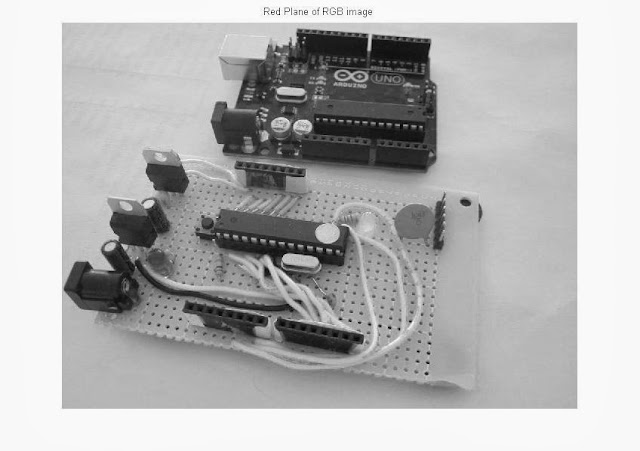# Using MATLAB, Extracting individual Red, Blue & Green (RGB) planes, From a RGB Image, With Code

Extraction of individual Red, Green & Blue from a RGB image is a simple task, just follow these steps if you are new to this process:

1. Suppose we are having an image known as 'xyz.jpg', which is a RGB image.

Now we are going to read that image in MATLAB, by using the command (if the image is in current working directory), imread('xyz.jpg'); or if the image is stored in other folder simply we have to provide the path of that image including the image.

Suppose the path of the image is 'E:\bunty\xyz.jpg' so we have to write like this imread('E:\bunty\xyz.jpg');.

Always prefer to create an exclusive variable for an image, for example here it will be x, x=imread('xyz.jpg');  The creation of a dedicated variable makes it easy to operate anything.
NOTE: Use ';' (Semicolon) to supress the output other wise you will see a large matrix of integers in front of you in the command window!
2. Now use the command imshow(x), in order to view the image just loaded as variable x.

3. Extraction of red (R) plane: We will be storing the red plane of the RGB image in the variable 'xR'. Therefore the command will be xR=x(:,:,1);, now you can use imshow(xR) in order to see the red plane of the RGB image.

4.  Extraction of green (G) plane: We will be storing the green plane of the RGB image in the variable 'xG'. Therefore the command will be xG=x(:,:,2);, now you can use imshow(xG) in order to see the green plane of the RGB image.

5.  Extraction of blue (B) plane: We will be storing the blue plane of the RGB image in the variable 'xB'. Therefore the command will be xB=x(:,:,3);, now you can use imshow(xB) in order to see the blue plane of the RGB image.

So the complete code will be like:
clc;
clear all;
close all;
figure, imshow(x);
title('Original RGB Image');
xR=x(:,:,1);
figure, imshow(xR);
title('Red Plane of RGB image');
xG=x(:,:,2);
figure, imshow(xG)
title('Green Plane of RGB image');
xB=x(:,:,3);
figure, imshow(xB)
title('Blue Plane of RGB image');

Snapshots:Original RGB ImageBlue Plane OF RGB ImageGreen Plane OF RGB ImageRed Plane OF RGB Image

1.1.2.